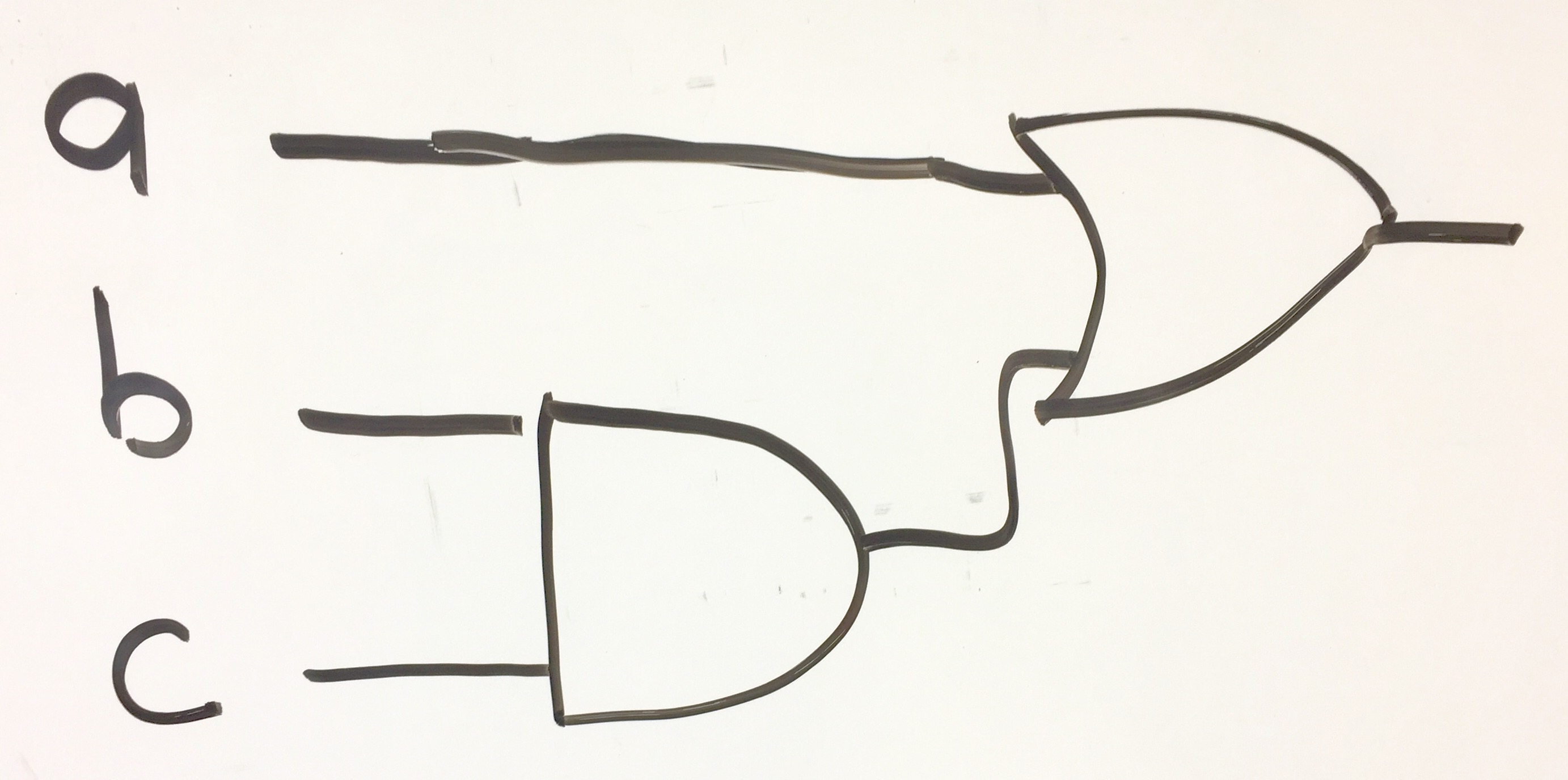## P1

Convert the following circuit into a 3CNF formula following our polynomial time recuction of CIRCUIT-SAT to 3CNF-SAT, as given in the notes.## P2

Consider the following instance of 3CNF-SAT: $F = (c \vee a) \wedge (c \vee b) \wedge (\neg a \vee \neg b \vee \neg c) \wedge (\neg c)$

1. Follow the reduction algorithm that reduces 3CNF-SAT to INDEPENDENT-SET, and draw the resulting graph (and k value).
2. Clearly show which vertices form the independent set of size $k$.
3. Clearly state the associated satisfying assignment for the variables in formula $F$.

## P3

Do counting sort to sort the array of (row,col) positions in increasing order by column. Show the "C" array of counts that is produced, as well as the array "C" after the summing step and, of course, show the sorted output array.
	(4,2), (4,0), (3,2), (2,4), (0,0), (1,2), (4,4)


## P4

Show what the parition algorithm would produce on the following input. Clearly state what k value would be return, and show what array A looks like after partition is done.
   ... 	d   c   j   b   f   a   h   i   e   g ...
---------------------------------------
... 12  13  14  15  16  17  18  19  20  21 ...